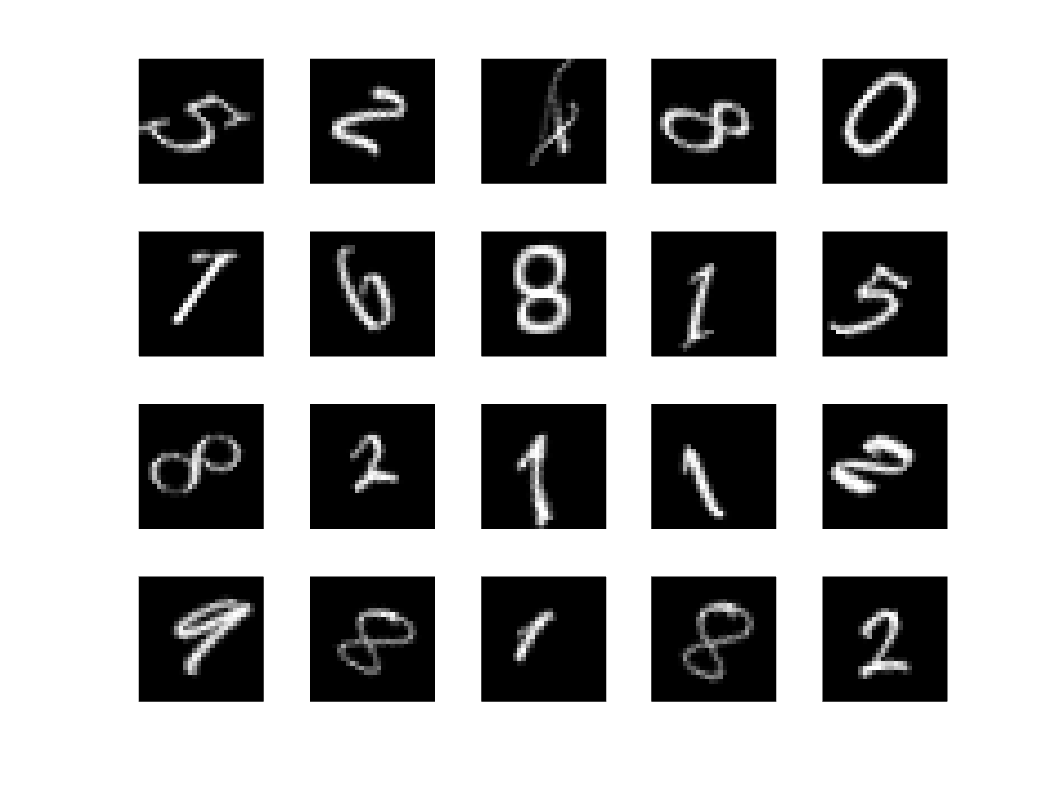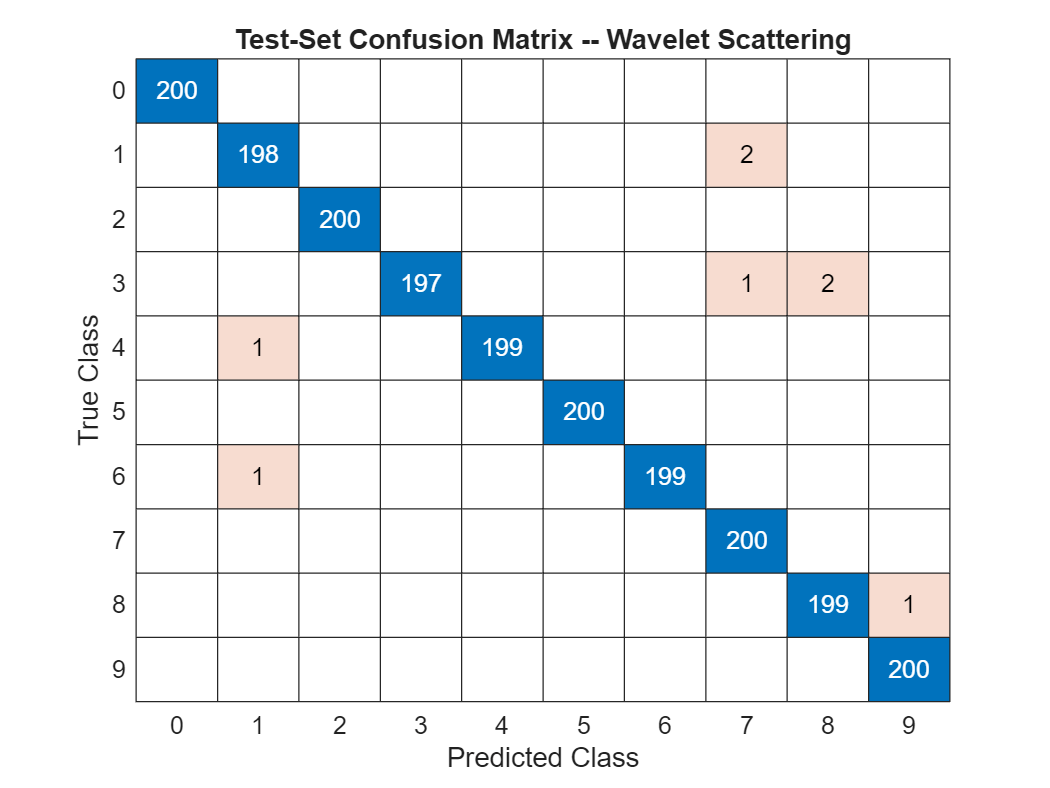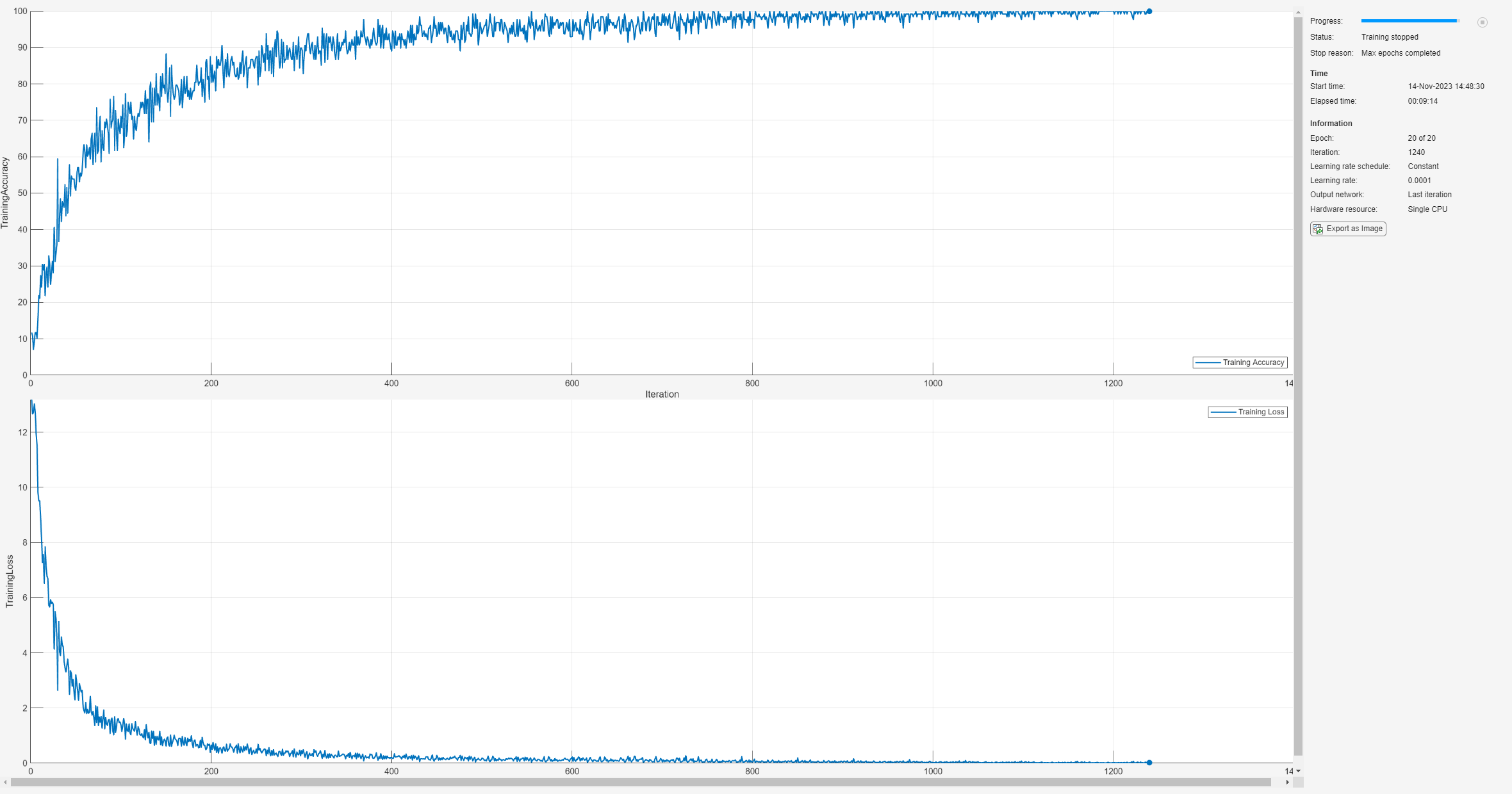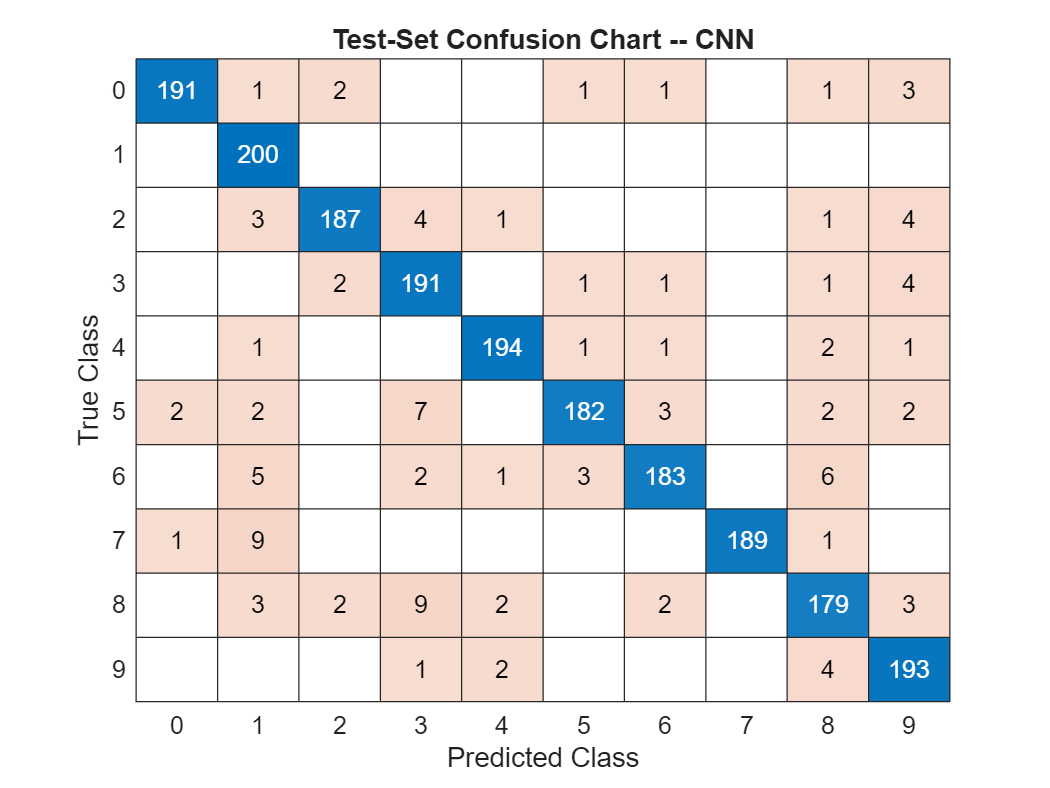Digit Classification with Wavelet Scattering

This example shows how to use wavelet scattering for image classification. This example requires Wavelet Toolbox™, Deep Learning Toolbox™, and Parallel Computing Toolbox™.

For classification problems, it is often useful to map the data into some alternative representation which discards irrelevant information while retaining the discriminative properties of each class. Wavelet image scattering constructs low-variance representations of images which are insensitive to translations and small deformations. Because translations and small deformations in the image do not affect class membership, scattering transform coefficients provide features from which you can build robust classification models.

Wavelet scattering works by cascading the image through a series of wavelet transforms, nonlinearities, and averaging . The result of this deep feature extraction is that images in the same class are moved closer to each other in the scattering transform representation, while images belonging to different classes are moved farther apart. While the wavelet scattering transform has a number of architectural similarities with deep convolutional neural networks, including convolution operators, nonlinearities, and averaging, the filters in a scattering transform are pre-defined and fixed.

Digit Images

The dataset used in this example contains 10,000 synthetic images of digits from 0 to 9. The images are generated by applying random transformations to images of those digits created with different fonts. Each digit image is 28-by-28 pixels. The dataset contains an equal number of images per category. Use the imageDataStore to read the images.

digitDatasetPath = fullfile(matlabroot,'toolbox','nnet','nndemos','nndatasets','DigitDataset');
Imds = imageDatastore(digitDatasetPath,'IncludeSubfolders',true, 'LabelSource','foldernames');

Randomly select and plot 20 images from the dataset.

figure
numImages = 10000;
rng(100);
perm = randperm(numImages,20);
for np = 1:20
subplot(4,5,np);
imshow(Imds.Files{perm(np)});
endYou can see that the 8s exhibit considerable variability while all being identifiable as an 8. The same is true of the other repeated digits in the sample. This is consistent with natural handwriting where any digit differs non-trivially between individuals and even within the same individual's handwriting with respect to translation, rotation, and other small deformations. Using wavelet scattering, we hope to build representations of these digits which obscure this irrelevant variability.

Wavelet Image Scattering Feature Extraction

The synthetic images are 28-by-28. Create a wavelet image scattering framework and set the invariance scale to equal the size of the image. Set the number of rotations to 8 in each of the two wavelet scattering filter banks. The construction of the wavelet scattering framework requires that we set only two hyperparameters: the InvarianceScale and NumRotations.

sf = waveletScattering2('ImageSize',[28 28],'InvarianceScale',28, ...
'NumRotations',[8 8]);

This example uses MATLAB™'s parallel processing capability through the tall array interface. If a parallel pool is not currently running, you can start one by executing the following code. Alternatively, the first time you create a tall array, the parallel pool is created.

if isempty(gcp)
parpool;
end
Starting parallel pool (parpool) using the 'local' profile ...
Connected to the parallel pool (number of workers: 6).

For reproducibility, set the random number generator. Shuffle the files of the imageDatastore and split the 10,000 images into two sets, one for training and one held-out set for testing. Allocate 80% of the data, or 8,000 images, to the training set and hold out the remaining 2,000 images for testing. Create tall arrays from the training and test datasets. Use the helper function helperScatImages to create feature vectors from the scattering transform coefficients. helperScatImages obtains the log of the scattering transform feature matrix as well as the mean along both the row and column dimensions of each image. The code for helperScatImages is at the end of this example. For each image in this example, the helper function creates a 217-by-1 feature vector.

rng(10);
Imds = shuffle(Imds);
[trainImds,testImds] = splitEachLabel(Imds,0.8);
Ttrain = tall(trainImds);
Ttest = tall(testImds);
trainfeatures = cellfun(@(x)helperScatImages(sf,x),Ttrain,'UniformOutput',false);
testfeatures = cellfun(@(x)helperScatImages(sf,x),Ttest,'UniformOutput',false);

Use tall's gather capability to concatenate all the training and test features.

Trainf = gather(trainfeatures);
Evaluating tall expression using the Parallel Pool 'local':
- Pass 1 of 1: Completed in 3 min 51 sec
Evaluation completed in 3 min 51 sec
trainfeatures = cat(2,Trainf{:});
Testf = gather(testfeatures);
Evaluating tall expression using the Parallel Pool 'local':
- Pass 1 of 1: Completed in 49 sec
Evaluation completed in 49 sec
testfeatures = cat(2,Testf{:});

The previous code results in two matrices with row dimensions 217 and column dimension equal to the number of images in the training and test sets respectively. Accordingly, each column is a feature vector for its corresponding image. The original images contained 784 elements. The scattering coefficients represent an approximate 4-fold reduction in the size of each image.

PCA Model and Prediction

This example constructs a simple classifier based on the principal components of the scattering feature vectors for each class. The classifier is implemented in the functions helperPCAModel and helperPCAClassifier. helperPCAModel determines the principal components for each digit class based on the scattering features. The code for helperPCAModel is at the end of this example. helperPCAClassifier classifies the held-out test data by finding the closest match (best projection) between the principal components of each test feature vector with the training set and assigning the class accordingly. The code for helperPCAClassifier is at the end of this example.

model = helperPCAModel(trainfeatures,30,trainImds.Labels);
predlabels = helperPCAClassifier(testfeatures,model);

After constructing the model and classifying the test set, determine the accuracy of the test set classification.

accuracy = sum(testImds.Labels == predlabels)./numel(testImds.Labels)*100
accuracy = 99.6000

We have achieved 99.6% correct classification of the test data. To see how the 2,000 test images have been classified, plot the confusion matrix. There are 200 examples in the test set for each of the 10 classes.

figure;
confusionchart(testImds.Labels,predlabels)
title('Test-Set Confusion Matrix -- Wavelet Scattering')CNN

In this section, we train a simple convolutional neural network (CNN) to recognize digits. Construct the CNN to consist of a convolution layer with 20 5-by-5 filters with 1-by-1 strides. Follow the convolution layer with a RELU activation and max pooling layer. Use a fully connected layer, followed by a softmax layer to normalize the output of the fully connected layer to probabilities. Use a cross entropy loss function for learning.

imageSize = [28 28 1];
layers = [ ...
imageInputLayer([28 28 1])
convolution2dLayer(5,20)
reluLayer
maxPooling2dLayer(2,'Stride',2)
fullyConnectedLayer(10)
softmaxLayer
classificationLayer];

Use stochastic gradient descent with momentum and a learning rate of 0.0001 for training. Set the maximum number of epochs to 20. For reproducibility, set the ExecutionEnvironment to 'cpu'.

options = trainingOptions('sgdm', ...
'MaxEpochs',20,...
'InitialLearnRate',1e-4, ...
'Verbose',false, ...
'Plots','training-progress','ExecutionEnvironment','cpu');

Train the network. For training and testing we use the same data sets used in the scattering transform.

reset(trainImds);
reset(testImds);
net = trainNetwork(trainImds,layers,options);By the end of training, the CNN is performing near 100% on the training set. Use the trained network to make predictions on the held-out test set.

YPred = classify(net,testImds,'ExecutionEnvironment','cpu');
DCNNaccuracy = sum(YPred == testImds.Labels)/numel(YPred)*100
DCNNaccuracy = 95.5000

The simple CNN has achieved 95.5% correct classification on the held-out test set. Plot the confusion chart for the CNN.

figure;
confusionchart(testImds.Labels,YPred)
title('Test-Set Confusion Chart -- CNN')Summary

This example used wavelet image scattering to create low-variance representations of digit images for classification. Using the scattering transform with fixed filter weights and a simple principal components classifier, we achieved 99.6% correct classification on a held-out test set. With a simple CNN in which the filters are learned, we achieved 95.5% correct. This example is not intended as a direct comparison of the scattering transform and CNNs. There are multiple hyperparameter and architectural changes you can make in each case, which significantly affect the results. The goal of this example was simply to demonstrate the potential of deep feature extractors like the wavelet scattering transform to produce robust representations of data for learning.

References

 Bruna, J., and S. Mallat. "Invariant Scattering Convolution Networks." IEEE Transactions on Pattern Analysis and Machine Intelligence. Vol. 35, Number 8, 2013, pp. 1872–1886.

 Mallat, S. "Group Invariant Scattering." Communications in Pure and Applied Mathematics. Vol. 65, Number 10, 2012, pp. 1331–1398.

 Sifre, L., and S. Mallat. "Rotation, scaling and deformation invariant scattering for texture discrimination." 2013 IEEE Conference on Computer Vision and Pattern Recognition. 2013, pp 1233–1240. 10.1109/CVPR.2013.163.

Appendix — Supporting Functions

helperScatImages

function features = helperScatImages(sf,x)
% This function is only to support examples in the Wavelet Toolbox.
% It may change or be removed in a future release.

smat = featureMatrix(sf,x,'transform','log');
features = mean(mean(smat,2),3);
end

helperPCAModel

function model = helperPCAModel(features,M,Labels)
% This function is only to support wavelet image scattering examples in
% Wavelet Toolbox. It may change or be removed in a future release.
% model = helperPCAModel(features,M,Labels)

% Initialize structure array to hold the affine model
model = struct('Dim',[],'mu',[],'U',[],'Labels',categorical([]),'s',[]);
model.Dim = M;
% Obtain the number of classes
LabelCategories = categories(Labels);
Nclasses = numel(categories(Labels));
for kk = 1:Nclasses
Class = LabelCategories{kk};
% Find indices corresponding to each class
idxClass = Labels == Class;
% Extract feature vectors for each class
tmpFeatures = features(:,idxClass);
% Determine the mean for each class
model.mu{kk} = mean(tmpFeatures,2);
[model.U{kk},model.S{kk}] = scatPCA(tmpFeatures);
if size(model.U{kk},2) > M
model.U{kk} = model.U{kk}(:,1:M);
model.S{kk} = model.S{kk}(1:M);

end
model.Labels(kk) = Class;
end

function [u,s,v] = scatPCA(x,M)
% Calculate the principal components of x along the second dimension.

if nargin > 1 && M > 0
% If M is non-zero, calculate the first M principal components.
[u,s,v] = svds(x-sig_mean(x),M);
s = abs(diag(s)/sqrt(size(x,2)-1)).^2;
else
% Otherwise, calculate all the principal components.
% Each row is an observation, i.e. the number of scattering paths
% Each column is a class observation
[u,d] = eig(cov(x'));
[s,ind] = sort(diag(d),'descend');
u = u(:,ind);
end
end
end

helperPCAClassifier

function labels = helperPCAClassifier(features,model)
% This function is only to support wavelet image scattering examples in
% Wavelet Toolbox. It may change or be removed in a future release.
% model is a structure array with fields, M, mu, v, and Labels
% features is the matrix of test data which is Ns-by-L, Ns is the number of
% scattering paths and L is the number of test examples. Each column of
% features is a test example.

labelIdx = determineClass(features,model);
labels = model.Labels(labelIdx);
% Returns as column vector to agree with imageDatastore Labels
labels = labels(:);

%--------------------------------------------------------------------------
function labelIdx = determineClass(features,model)
% Determine number of classes
Nclasses = numel(model.Labels);
% Initialize error matrix
errMatrix = Inf(Nclasses,size(features,2));
for nc = 1:Nclasses
% class centroid
mu = model.mu{nc};
u = model.U{nc};
% 1-by-L
errMatrix(nc,:) = projectionError(features,mu,u);
end
% Determine minimum along class dimension
[~,labelIdx] = min(errMatrix,[],1);

%--------------------------------------------------------------------------
function totalerr = projectionError(features,mu,u)
%
Npc = size(u,2);
L = size(features,2);
% Subtract class mean: Ns-by-L minus Ns-by-1
s = features-mu;
% 1-by-L
normSqX = sum(abs(s).^2,1)';
err = Inf(Npc+1,L);
err(1,:) = normSqX;
err(2:end,:) = -abs(u'*s).^2;
% 1-by-L
totalerr = sqrt(sum(err,1));
end
end
end